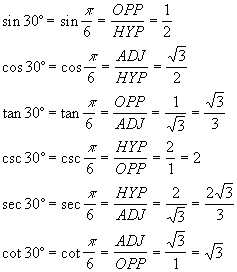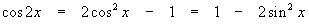Basics · Identities · Examples · Formulae · Exercizes · Advanced
Keywords · Navigation · Recommended Books · Site Index · Trig Index · Gift Shop

###Trigonometry: The very fast review

I. BasicsTrigonometry is nothing more than how to deal with angles versus straight lines. The tricky part comes because the numbers instantly get very messy and hard to handle. Mathematics is exact by default, so we cannot cheat by clipping off digits when we no longer feel like writing them down. We have to have a decent way to handle ugly numbers. The most trigonometric way to do this is to look at several example triangles (which have “almost” nice numbers):Example 1. The 45° triangleFigure 1- The 45° triangle

According to Pythagorus,. Using the definitions of the trig functions gives usExample 2. The 30°/60° triangleFigure 2 - The 30°/60° triangle

According to Pythagorus,. Using the definitions of the trig functions givesExample 3. The 15°/75° triangleFigure 3 - The 15°/75° triangle

Now we make use of the formulasAddition , SubtractionThere are other triangles, but I am lazy. Let's see how far you can get with just those I've done so far and a few simple laws (so you can add and subtract and multiply and divide angles). Please note that getting 1/3 of an angle is equivalent to trisecting it, a classic impossible problem! ( Advanced - because the answer depends on solving a cubic. The answer is rather dirty-looking, anyway).Double & half angles

And there are as many more as you care to memorize (always a mistake!). The hard part will be in coming up with smaller divisions (such as 5 degrees) without the arithmetic getting infinitely hairy. The author tried for hours and gave up!

II. Trig IdentitiesThe only two trig functions worth worrying about are sin x and cos x ; all others are derived from these. Imagine a circle of radius r = 1 centered at the origin of the x, y plane (see Figure 1) and a point anywhere on that circle.Figure 4 - The Unit Circle

Call the angle counterclockwise from the x-axis , the x-coordinate of the point x and the y-coordinate y. Then, with r as the longest leg of the resulting triangle, we clearly have a right triangle, so according to PythagorusBy definition,so, remembering to set r = 1, we havePythagorus' Law, Trig version

The rest of the common trig functions come from these definitions:From these, we can find literally tons of “cool” trig identities: For example,soorPythagorus in terms of sec and tan

On the other hand,soPythagorus in terms of csc and cot

If you don't mind messing with exponentials and imaginary numbers, you can easily derive the sum rule for cosine:which proves the familiarcosine sum rule

If you have followed me so far, then we can take the last result and find out that, ifthendouble angle formula.

Also, becausewe also have bothDouble angle formulas

This provides us an opportunity to sayfrom which we get the first of the half-angle formulasSine half-angle formula

Once you get the rules in trig form (as opposed to exp form, complicated and unfamiliar!), you can keep going until you are asleep: Let's assume that we provedSine sum rule

the same way as the cosine relationship above (you do it for practice if you need to know how to manipulate those exponentials) and derive the similar formula for tangent:this givesTangent sum rule

There are several really good tricks to remember, they all look different in trig than they do in algebra:Difference between two squares

see the formula page for the rest - all algebra tricks apply directly to trig (with some cautions!).Recommended Books

The classic trigonometry problem book - very light on theory, plenty of problems with full solutions, more problems with answersKeywords

trigonometry, angle, sine, cosine, triangle, tangent, cotangent, cosecant, secant